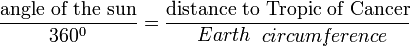Sal's RuneScape Forum# Eratosthenes and some Circumference

## Recommended Posts

More than 2200 years ago, the Greek mathematician Eratosthenes used similar triangles to estimate the circumference of the earth. He got the idea when he observed that at noon on the day of the summer solstice, the sun shone straight down to the bottom of the well in the town of Syene(which is called Aswan, Egypt, today).

a) Find out how Eratosthences solved the problem and explain why his solution was a valid way to estimate the size of the Earth.

b) List some limitations of his method.

Minimum 1 page

Use diagrams, pictures, etc.

Show that you learned something

You will find this same question on google but this is out of my textbook so yeh.

I've read like 10 pages that explain this but I just don't get it. ^.^

Its driving me nutssssssssssssss

##### Share on other sitesMeasurements taken at Alexandria (A) and Syene (S)

that diagram is rather explanatory of how he did it

the reason why the sun is at its zenith over the city of Syene is because it is located on the tropic of cancer where this can occur on the summer solstice. while he would have measured an angle of elevation of 0 degrees in Syene as the sun was at its zenith, in Alexandria at the same time on the summer solstice the angle of elevation was 7°12' which is 1/50th of a circle. as the degree measurement was 1/50th of a circle, the arc's measurement was 1/50th of the earth's circumference. he figured out the arc measurement from the known distance between the two cities (5000 stadia or 927.7 km), and then figured that every degree in the circumference is equal to estimated 700 stadia from this. then 700 x 360 is 252,000 stadia or approx 39,690 km (error < 2%, as actual circumference is 40,075 km)

diagram of it including calculationsyou can also figure out the diameter/radius of the earth by using these results, as if the circumference is calculated as 39,690 km then:

39690 = 2πr

r = 6,316.86 km

6316.86 x 2 = d

d = 12,633.72 km

Edited by Clavius

##### Share on other sites

One more thing.

Where are the similar triangles? :o

Thats what the whole point of this is. ^.^

I don't understand wiki.

Edit:OH its due tomorrow.

For b) I put...

The measurement b/t the cities wasn't accurate. Right?

Edited by Aabid

##### Share on other sites

Might as well already tell you if you still don't know. Two similar triangles have the same angles, but different length legs. So if two triangles have 30, 60, 90 degree angles, but one is 4 meters tall and the other is 2 meters tall, they're similar. You can do direct ratios using them.

##### Share on other sites

One more thing.

Where are the similar triangles? :o

Thats what the whole point of this is. ^.^

I don't understand wiki.

Edit:OH its due tomorrow.

For b) I put...

The measurement b/t the cities wasn't accurate. Right?

the measurement wasn't accurate due to the somewhat crude nature of both measuring the angle of elevation and the distance between the two cities. it is rather accurate however, as it was the first measure of the circumference of the earth ever done... you could replicate this experiment today, it just requires good timing and a small amount of planning.

two other interesting things you could estimate from the results are the volume and surface area of the earth, all you have to do is assume that the earth is a sphere which gives a decent amount of error but still rather good for so long ago (not sure when Eratosthenes did this, but if it was after 225 BC he would have had the formulas proven by Archimedes and been able to calculate the surface area at least).

the surface area from the given radius is 487,009,391 sq km (real value 510,100,000 sq km so error of 4.5%)

volume from given radius is 1,055,394,856,531 cu km (real value 1,083,210,000,000 so error of 2.6%)

Edited by Clavius

##### Share on other sites

Might as well already tell you if you still don't know. Two similar triangles have the same angles, but different length legs. So if two triangles have 30, 60, 90 degree angles, but one is 4 meters tall and the other is 2 meters tall, they're similar. You can do direct ratios using them.

Yessir know this.

Where are the triangles in this diagram?Is this right? ^.^

Also, I really need limitations. I wrote that the measurement b/t cities could have been wrong.

One moree thing.

I see the similar angles

7 degrees.

Now where are the other proportioned measurements?7.2/360=????

Edit:Made it again.

Edited by Aabid

##### Share on other sites

To make this work you need a place where the sun's rays are perpendicular to the ground. That doesn't happen always nor does it happen everywhere, you need to be between the tropic of cancer and the tropic of Capricorn for that to happen. And if you look at the world map, that's not a big area...So that's a small limitation...

But this is a bit weird... According to wikipedia, Syene lies 24° north while the tropic of cancer is 23.5° north. Which means that either the story is wrong, Syene or the place where he did the actual measurement was a bit more south or 2200 years ago the tropic of cancer was a bit more to the north (it does change over time).

## Create an account

Register a new account